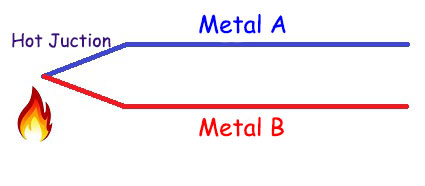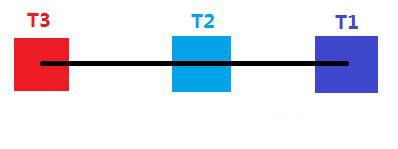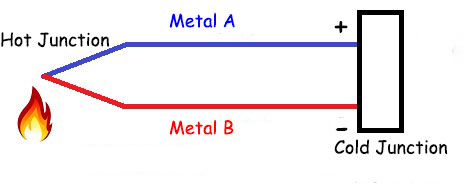Close
(0) Shopping cart
You have no items in your shopping cart.
Shopping Categories
Search

# Thermocouple Working Principle

Thermocouple temperature sensors are characterized by wide range, low cost, fast response and durability. They are widely used for temperature measurement in industry. R-type thermocouples can measure high temperatures of more than 1700 degrees, and are mainly used in high temperature measurement occasions. In today's article, let's talk about how thermocouples work.

thermocouple is a sensor that connects two ends of metals of different materials and uses the thermoelectric effect to measure temperature. As shown below:Thermocouples are based on the thermoelectric effect. Then what is the thermoelectric effect?

As we know, when a voltage is applied across a length of wire, current flows through the wire and heats up. This phenomenon is called the thermal effect of current. In 1821, German scientist Thomas Johann Seebeck discovered the inverse effect of the thermal effect of current. When different temperatures are applied to both ends of a piece of wire, electromotive force will be generated at both ends of the wire. After closing the loop, a current will flow through the wire. This phenomenon is called the thermoelectric effect, also known as the Seebeck effect. Below is an illustration of the thermoelectric effect:The black line in the figure represents the metal wire, T1, T2 and T3 represent different temperature differences on the metal wire, and T3 is greater than T1. Assuming that the temperature difference between T3 and T1 is ΔT, the voltage across the wire is:

V31=S(T)xΔT, where S(T) is the Seebeck coefficient.

Why would a thermocouple use two metals when a voltage difference can be created across a wire at different temperatures? The answer is: If the probe of the voltmeter is of the same metal as the wire being measured, the voltmeter will not measure any voltage theoretically. Since it is equivalent to extending the wire, and there is no temperature difference between the two ends of the extended wire, so there is no voltage difference.

Seebeck coefficients are different for different metals, and the measurement voltage is equal to the integral of the difference between Seebeck coefficient functions of the two materials, that is why thermocouples use two different metals. According to the type and content of the two metals, thermocouples can be divided into different types:

 Type Composition Temperature Range T copper / constantan -250°C to 400°C J iron / constantan -180°C to 750°C E chromel / constantan -40°C to 900°C K chromel / alumel -180°C to 1,200°C S platinum-rhodium (10%) / platinum 0°C to 1,700°C R platinum-rhodium (13%) / platinum 0°C to 1,700°C B platinum-rhodium (30%) / platinum-rhodium (6%) 0°C to 1,800°C N nicrosil / nisil -270°C to 1,280°C

The junction of the two metals in the thermocouple is called measuring junction, also known as hot junction. The corresponding one is called cold junction. The cold junction is the reference, and ice water temperature (0°C) was used as a reference in the early days. Cold junction as a reference, the temperature of the hot junction is calculated from the measured voltage.

With the development of technology, the cold junction of the thermocouple doesn’t have to be 0°C. At present, with the help of cold junction compensation technology, some PLC thermocouple modules can calculate the temperature of the hot junction according to the measured voltage value. The schematic diagram is as follows:Well, so much for the working principle of thermocouples. If you like this article, please leave us a comment.